Stephen Muirhead meets neither, as he explores waves, tiles and percolation theoryWhile catching up on past issues of Chalkdust over the Christmas break, I was intrigued to see that issue 07 featured an article on Chladni figures (On the cover: Chladni figures of a square drum by James Cann), followed in issue 08 by an article on Truchet tiles (Too good to be Truchet by Colin Beveridge). This coincidence fascinated me because, although not the focus of the articles, there is a remarkable conjecture that links these two apparently unrelated objects, via the mathematics of percolation theory.

## Chladni figures and random waves

As explained in issue 07, Chladni figures are the complex nodal patterns created by the zero-set of standing waves, illustrated most famously by Ernst Chladni in his 18th-century experiments with sand sprinkled on a vibrating plate.

Chladni figures are of interest in many different domains of mathematics and science (for example, in quantum mechanics, oceanography, astrophysics etc). One problem faced in applications is that, as the frequency of the standing waves gets larger, the Chladni figures get more and more intricate and chaotic. A useful approximation is to consider randomised versions of standard waves, but this still raises the question of how to understand and describe the zero-set of these random waves (which we can think of as random Chladni figures).

## Random Truchet tilingsThe two kinds (up to rotation) of square Truchet tiles and the three kinds (up to rotation) of hexagonal Truchet tiles.

As introduced in issue 08, Truchet tiles are a set of polygonal tiles, which can be square, hexagonal, or some other shape, coloured black and white such that the corners are alternating in colour. These tiles can be arranged in various ways to create complex and beautiful tilings, and were first described in the 18th-century by French polymath and priest Father Sébastian Truchet.

In issue 08, the combinatorics of these tiles were considered, but it turns out that they are a useful tool with which to understand Chladni figures. In fact, there is an amazing conjecture, due to the physicists Bogomolny and Schmit in 2002, that says that random Chladni figures look identical, on large scales, to the patterns created by a random tiling of square Truchet tiles. To form this tiling, we start with a square lattice (or grid) and assign to each vertex a small disk coloured black or white in an alternating pattern.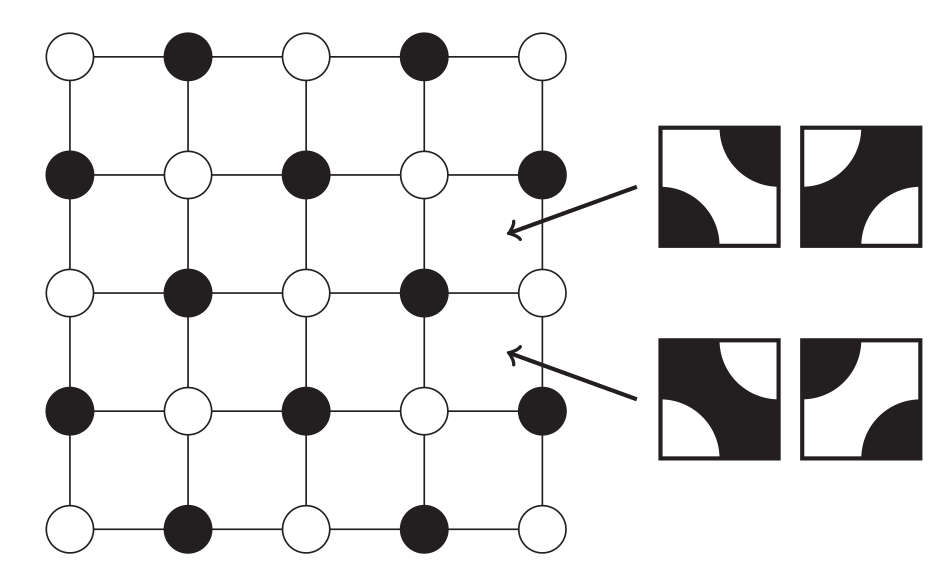The square lattice with alternating coloured disks and the two kinds of square Truchet tiles that are compatible with each face of the lattice.

Notice that, for each face of the square lattice, there are two possible square Truchet tiles that can be placed whose colours are compatible with the disks. Choosing these two possibilities independently at random with equal probability (which has the appealing property of retaining black/white symmetry), we have created a random Truchet tiling of the entire plane. The Bogomolny–Schmit conjecture says that the statistical properties of the interfaces of the tiling (ie the curves that separate the black and white regions) match, on large scales, the statistical properties of the curves created by random Chladni figures. To be concrete, examples of such large-scale statistical properties are the crossing probabilities, that is, the probability that a curve crosses a large rectangle from left-to-right.

Where does this conjecture come from? The reasoning is actually quite simple. We first observe that the local maxima of standing waves are all positive, and the local minima are all negative (mathematically, this is a consequence of the standing waves being solutions to the Helmholtz equation). Then we suppose that a random standing wave has roughly as many local maxima as local minima and that these are arranged in a square lattice, with saddle points between every pair of maxima/minima. Finally, we assume that the saddle points take positive or negative values independently with equal probability; using the sign of the saddle points to connect up neighbouring maxima/minima, and identifying $+$’ with black and $-$’ with white, this yields a model for random Chladni figures that is equivalent to the random Truchet tiling.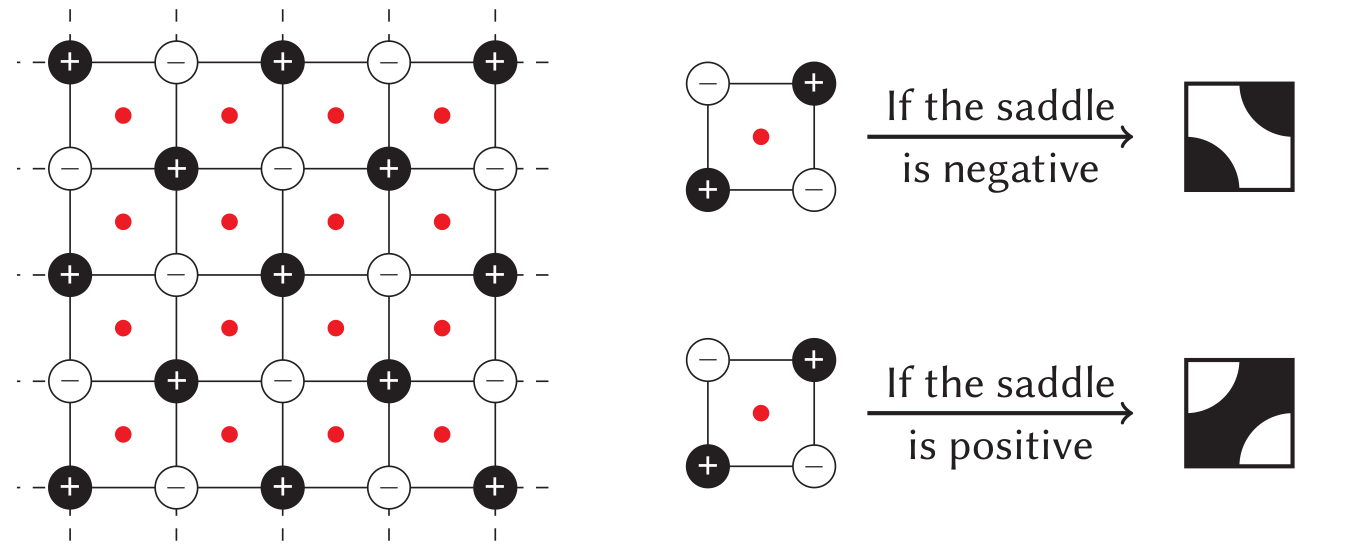A grid of maxima/minima/saddles, and replacement rules to form the random Truchet tiling.

So does this conjecture help us understand Chladni figures? Doesn’t it just tell us that one complicated object—a typical high-frequency Chladni figure—is well-approximated by another complicated object—a random Truchet tiling? Well yes, but this is actually an immense improvement, because in fact random Truchet tilings are just a thinly-disguised version of a well-understood object in probability theory—critical percolation.

## Truchet tilings as a model for percolation

Percolation is the mathematical study of porous media, like sandstone or Swiss cheese. There are many models of percolation, but perhaps the simplest is to start with an infinite periodic lattice, such as the square or hexagonal lattice, and colour every edge of the lattice black or white independently with respective probabilities $p$ and $1-p$ for some fixed $p \in [0,1]$ (this model is called bond or edge percolation; one could alternatively colour every face black or white, which is called face percolation, or every vertex black or white, which is called site percolation). One then considers the subgraph formed by the black edges, which is a simple model for an inhomogeneous porous medium.

There are two crucial facts about percolation. The first is that, for essentially any model of percolation, there is a critical probability $p_c$ such that if $p < p_c$ then the black edges form only finite clusters (ie finite connected subgraphs), whereas if $p > p_c$ then there is a black cluster that is infinite. In other words, there is a phase transition in the global connectivity of the model, from a graph that is composed of finite clusters, to a graph that is globally connected (although it will also contain some small disjoint clusters).

The second fact is that, whereas certain local features of percolation depend heavily on the particular percolation model being considered, many of the large-scale properties of the model are universal, that is, they behave in essentially the same way in the three regimes $p < p_c$, $p=p_c$ and $p>p_c$ for every percolation model. Examples of local or non-universal properties include the value of $p_c$ itself, and also the number of black clusters contained in a given large region, whereas examples of universal properties include the crossing probabilities mentioned earlier. Understanding the universal properties of percolation is an active area of research, especially in the critical regime $p=p_c$, and two Fields medals have been recently awarded for progress in this area (Wendelin Werner in 2006 and Stanislav Smirnov in 2010).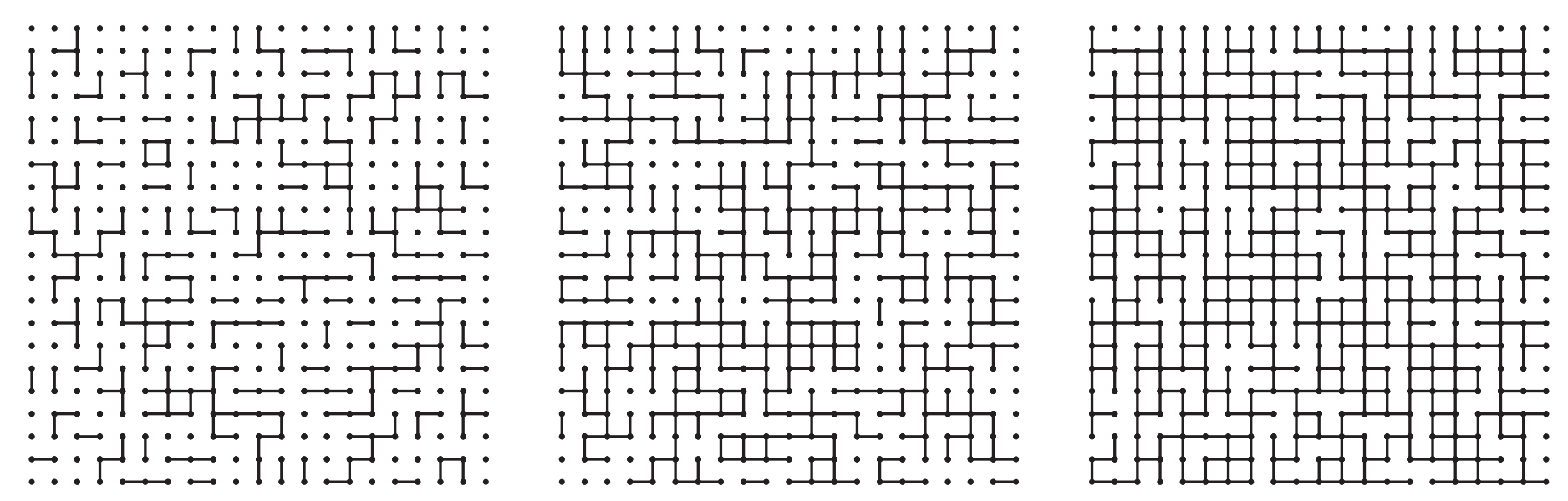Bond percolation on the square lattice in the sub-critical ($p < p_c$), critical ($p = p_c$) and super-critical ($p > p_c$) regimes. For this model $p_c = 1/2$.

Let’s make the link to random Truchet tilings. As we indicated, the random Truchet tiling is just a disguised version of bond percolation on the square lattice with parameter $p=1/2$. To see why, start with the percolation model, rotate the lattice by 45°, draw a red vertex in the centre of every face, and then replace the black and white edges with the corresponding Truchet tile so that the models coincide.Bond percolation on the square lattice, a rotated version, and the replacement rules that create the corresponding Truchet tiling.

So which percolation regime does the random Truchet tiling correspond to? It turns out that the critical probability for bond percolation on the square lattice is precisely $p_c = 1/2$ (this can be understood, in terms of the Truchet tiling, as a consequence of the black/white symmetry), so the random Truchet tiling should behave on large scales like critical percolation. Hence if the Bogomolny–Schmit conjecture is true, random Chladni figures also behave, on large scales, exactly like critical percolation. Since the properties of critical percolation are well-understood, this gives us a way to analyse, and make predictions about, the more complicated random Chladni figures.

## So is the Bogomolny–Schmit conjecture true?

There are a few aspects of the reasoning of Bogomolny–Schmit that are hard to swallow. In particular, the argument relied on the assumption that the local maxima/minima of a random standing wave are arranged in a square grid, which is simply not true. So it is natural to be sceptical.

While there hasn’t been much (to be fair, barely any!) mathematical progress on proving or disproving the conjecture, numerical studies (by Belyaev, Kerata, Nastasescu and others) have shed some light on the situation. What they show is that, for the non-universal features of critical percolation, the conjecture is wrong. This is unsurprising, since non-universal features should, by definition, depend on the specifics of the model (such as the choice of the square lattice over the hexagonal lattice), and as we just said, there are reasons to believe these specifics are not wholly accurate.

On the other hand, numerical studies have confirmed that the universal properties of critical percolation do match exactly the equivalent properties for random Chladni figures. This is remarkable, and it remains a genuine mystery why it is true.

## A percolation model with hexagonal Truchet tiles

In issue 08, different shapes of Truchet tiles were discussed, and we can easily generalise our random Truchet tiling to other shapes. Let’s consider the case of hexagonal tiles. Starting with a hexagonal lattice, we again assign to each vertex a small disk alternating black and white. There are now five choices of Truchet tiles that we can place in each hexagonal face that are compatible with the disks.

What probability should we assign to each choice of tile to get a sensible model for a random tiling? Well, since three of the five tiles are rotationally equivalent, it would be natural to assign them an equal probability $p$. To get a critical percolation model we also want to impose black/white symmetry, so the remaining two tiles should have equal probability $q=(1-3p)/2$. Since each of these probabilities depends on a single parameter $p \in [0,1]$, this defines a one-parameter family of percolation models, all of which will be critical.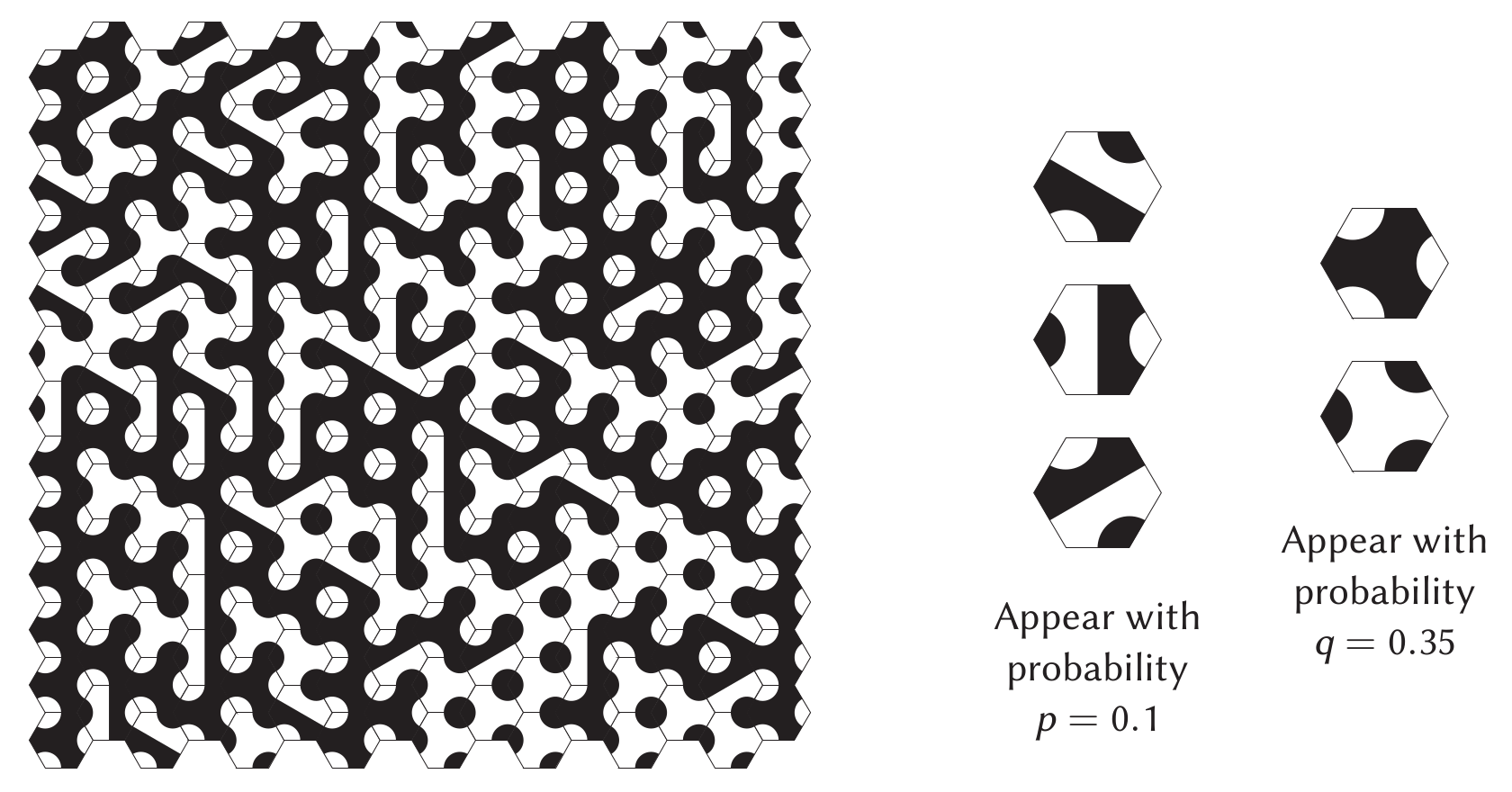Depiction of a large-scale random hexagonal tiling and a key to indicate which value of $p$ in the one-parameter family of models is being depicted.

In his seminal work in 2006, Smirnov considered the critical percolation model in which the faces of a hexagonal lattice are coloured black and white with equal probability; by exploiting the symmetries of the hexagonal lattice, he was able to establish rigorously, for the first time, many of the conjectured properties of critical percolation. Notice that this model is really just a degenerate case of the hexagonal Truchet tilings we constructed above, corresponding to the case $p=0$, and hence $q=1/2$ (to see this, simply remove the black/white circle segments from the Truchet tiles, which doesn’t affect the connectivity of the model). A few years later Chayes and Lei studied exactly the one-parameter family of critical hexagonal Truchet tilings defined above, and showed that they could also be studied with Smirnov’s techniques. It is a surprising fact that, 13 years after Smirnov’s work, these are (as far as I know) still the only critical percolation models which have been rigorously studied in detail. In other words, hexagonal Truchet tilings seem to possess a special structure that is unique among the (enormous!) class of critical percolation models.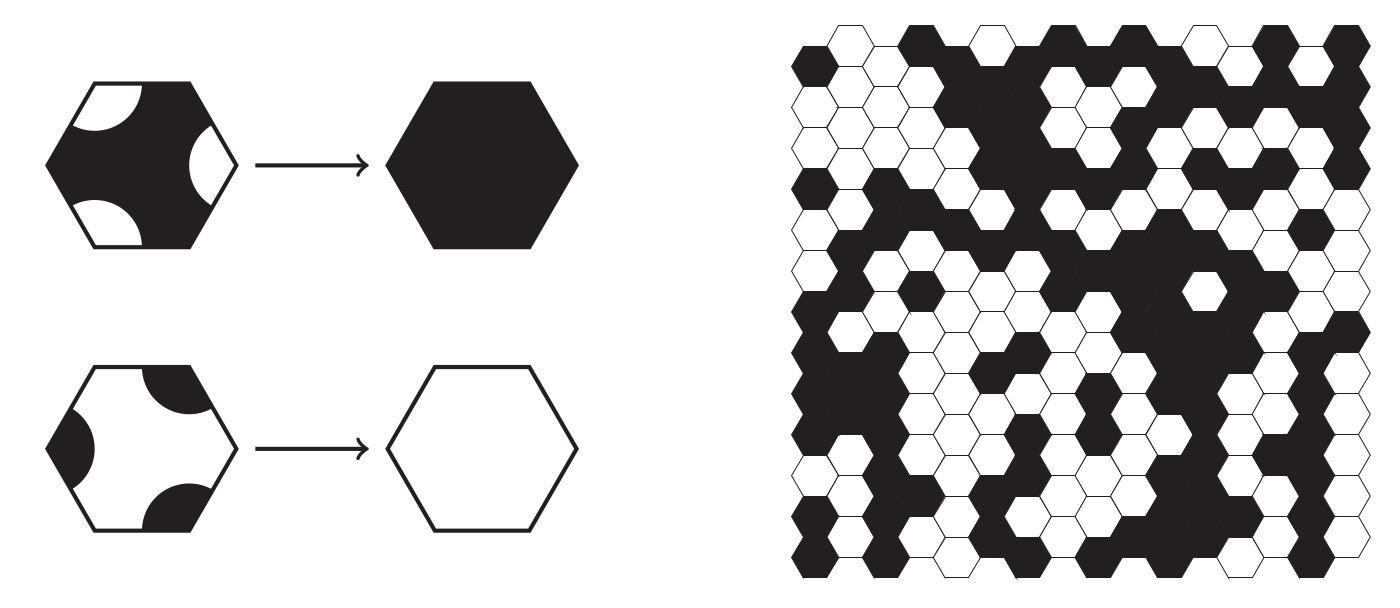Smirnov considered the percolation model using the faces of the hexagonal lattice rather than hexagonal Truchet tiles. In this model, the conjectured properties of critical percolation have been established.

## So did Truchet ever meet Chladni?

Let’s conclude by indulging in some idle speculation. Sébastian Truchet died in 1729, 27 years before the birth of Ernst Chladni in 1756, so they never had the opportunity to meet. However, as it turns out, Chladni was not the first scientist to investigate nodal patterns of standing waves—this was done half a century earlier by English scientist Robert Hooke. So did Truchet ever meet Hooke? Given their standing in the scientific communities of Paris and London (in 1700, Truchet and Hooke were simultaneously members of the Académie des sciences and the Royal Society respectively), it is quite possible that they did.Stephen is a lecturer in mathematics at Queen Mary University of London. Like any mathematician, he is always delighted to find unexpected connections between mathematical objects.
stephenmuirhead.wordpress.com    + More articles by Stephen